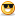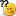# Thread: Geting data from odd rows of an excel sheet,

1. ## Geting data from odd rows of an excel sheet,

If i am in the database testing with wirnunner tool,
if i want to the get the data only from the odd rows of an excel sheet,
What is the statements i have to add to the TSL?
Pls give with an example.Reply With Quote

2. ## Re: Geting data from odd rows of an excel sheet,

Just a small logical change is needed to solve this problem.

Eg:
******************
for(i=1;i<=n;i++)
{
ddt_set_row(t,i);
set_window ("Inputs", 2);
a=ddt_val(t,"I1");
b=ddt_val(t,"I2");
edit_set("ThunderTextBox",a);
edit_set("ThunderTextBox_1",b);
button_press ("Ok");
edit_check_info("ThunderTextBox_2","value",a+b);
}
******************
This 'for' loop takes values from 2 columns of Excel sheet (I1 and I2) and adds them in the application after clicking 'OK' button. Then it checks the application output (Actual, 'value') with Expected (a+b).

Now, here if you want WinRunner to get the data only from odd rows of the Excel sheet then just change counter increment part of 'for' statement. It can be changed as below:

for(i=1;i<=n;i=i+2)This 'for' loop is executed with values of 'i' in the following series
1,3,5,7,9..........................

This is one way to get what you want. There are many more ways.Reply With Quote

3. ## Re: Geting data from odd rows of an excel sheet,

for(i=1;i<=n;i=i+2)
{
ddt_set_row(t,i);
set_window ("Inputs", 2);
for (j=1;j<=255;j++)
{
a=ddt_val(t,"j");
b=ddt_val(t,"j+1");
edit_set("ThunderTextBox",a);
edit_set("ThunderTextBox_1",b);
button_press ("Ok");
edit_check_info("ThunderTextBox_2","value",a+b);
}
}

the above for loop will add odd rows of the columns from 1 to 256. (as the excel has 256 columns)Reply With Quote

4.

####Posting Permissions

• You may not post new threads
• You may not post replies
• You may not post attachments
• You may not edit your posts
•